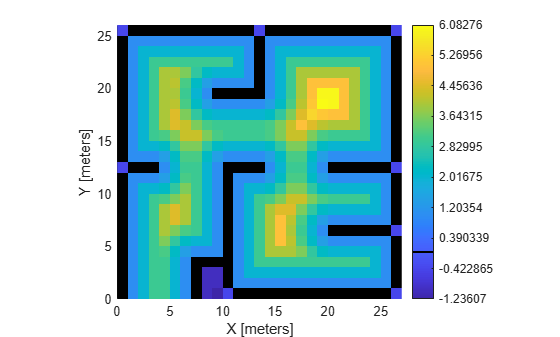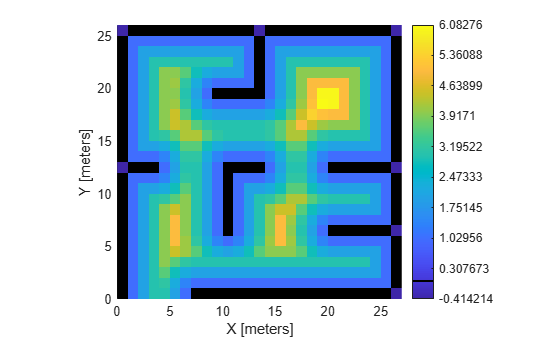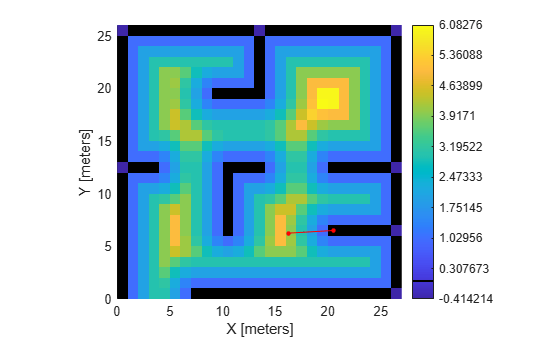# show

Display signed distance map

Since R2023a

## Syntax

``show(map)``
``show(map,frame)``
``show(___,Name=Value)``
``mapimage = show(___)``
``[mapimage,colorbar] = show(___)``

## Description

````show(map)` displays the occupancy grid `map` in the current axes, with the axes labels representing the world coordinates.```
````show(map,frame)` displays the occupancy grid `map` in the current axes, with the axes labels representing the coordinates in the coordinate frame `frame`.```

example

````show(___,Name=Value)` specifies additional options specified by one or more name-value arguments.```
````mapimage = show(___)` returns the handle to the image object `mapimage`, created by `show`.```
````[mapimage,colorbar] = show(___)` returns the handle to the colorbar `colorbar`, created by `show`.```

## Examples

collapse all

Load the `exampleMaps` MAT file.

`load exampleMaps.mat`

Create a signed distance map using the `simpleMap` data.

```sdm = signedDistanceMap(simpleMap,InterpolationMethod="none"); show(sdm,BoundaryColor=[0 0 0],Colorbar="on");``````setMapData(sdm,[7 1],zeros(5,5)) getMapData(sdm,[9 2])```
```ans = logical 0 ```
`show(sdm,BoundaryColor=[0 0 0],Colorbar="on");`Find the closest boundary to the coordinate, `[16.25 6.25]` and calculate the distance to the nearest boundary.

```coord = [16.25 6.25]; boundary = closestBoundary(sdm,coord,"world")```
```boundary = boundary(:,:,1) = 20.5000 boundary(:,:,2) = 6.5000 ```
`dist = distance(sdm,coord)`
```dist = 4 ```

Plot the line between the queried point and the closest obstacle cell center

```hold on plot([coord(1) boundary(:,:,1)],[coord(2) boundary(:,:,2)],"-r",Marker=".",MarkerSize=10)```Note that even though distance from the queried point appears greater than `4` when plotted, the distance function calculates the distance from the nearest cell center of the queried point.

## Input Arguments

collapse all

Signed distance map, specified as a `signedDistanceMap` object.

Coordinate frame, specified as either `"local"` or `"grid"`.

### Name-Value Arguments

Specify optional pairs of arguments as `Name1=Value1,...,NameN=ValueN`, where `Name` is the argument name and `Value` is the corresponding value. Name-value arguments must appear after other arguments, but the order of the pairs does not matter.

Example: `signedDistanceMap(sdm,Parent=ax)`

Axes to plot the map specified as either an `Axes` or `UIAxes` object. See `axes` or `uiaxes`.

Colormap values for the pixel values in the map, specified as a three-column matrix of RGB triplets. If not provided, the current colormap of the axes is used

Occupied boundary color, specified as an RGB triplet. The occupied boundary cells use the specified color when specified.

Use colorbar, specified as a logical `1` (`true`) or `0` (`false`). When `true`, `show` creates a colorbar that corresponds to the `Colormap` input and adds it to a hidden axis behind the current axis.

Data Types: `logical`

Vector field display type, specified as one of these options:

• `"off"` — Show no vector field.

• `"Gradient"` — Show gradient field superimposed on the distance map.

• `"ClosestBoundary"` — Show arrows that point to the nearest occupied boundary cell.

Data Types: `char` | `string`

## Output Arguments

collapse all

Map image handle, specified as a `image` object.

Colorbar handle, returned as a `colorbar` object.

## Version History

Introduced in R2023a